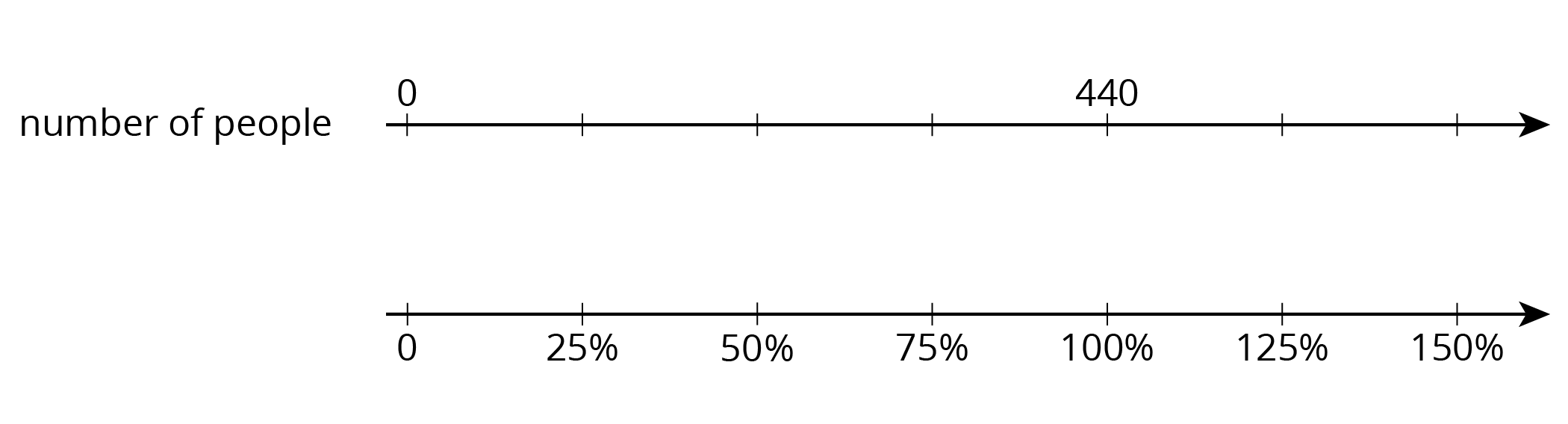# Mathematics Support

Math 6 | Unit 1 2 3 4 5 6 7 8 9

The Burj Khalifa

Rates

Percentages

## The Burj Khalifa

If you weighed four objects in pounds, then weighed the same four objects in kilograms, you might come up with this table.

weight (pounds) weight (kilograms)
22 10
88 40
33 15
40.7 18.5

Students are using what they know about ratios and rates to reason about measurements in different units of measurement such as pounds and kilograms. In earlier grades, students converted yards to feet using the fact that 1 yard is 3 feet, and kilometers to meters using the fact that 1 kilometer is 1,000 meters. Now in grade 6, students convert units that do not always use whole numbers.

Explain your strategy for each question.

1. Which is heavier, 1 pound or 1 kilogram?
2. A canoe weighs 99 pounds. How many kilograms does it weigh?
3. A watermelon weighs 12 kilograms. How many pounds does it weigh?

Solution:

Any correct strategy that your student understands and can explain is acceptable. Sample strategies:

1. 1 kilogram is heavier than 1 pound. When we weigh the same object in pounds and kilograms, the number of pounds is more than the number of kilograms. It takes fewer kilograms to express the weight of the same object, so each kilogram must be heavier than each pound. Another example of this idea: if we measure the length of a table in both meters and inches, the number of inches is more than the number of meters. Therefore, 1 inch must be shorter than 1 meter.
2. 45. Using the table, we can reason that 11 pounds is 5 kilograms. Multiplying each of these by 9 shows that 99 pounds is 45 kilograms.
3. 26.4. Using the table, we can find that each kilogram is equal to about 2.2 pounds. This means if we know an object’s weight in kilograms, we can multiply by 2.2 to find its weight in pounds. $12 X (2.2)=26.4 ## Rates Who biked faster: Andre, who biked 25 miles in 2 hours, or Lin, who biked 30 miles in 3 hours? One strategy would be to calculate a unit rate for each person. A unit rate is an equivalent ratio expressed as something “per 1.” For example, Andre’s rate could be written as “1212 miles in 1 hour” or “1212 miles per 1 hour.” Lin’s rate could be written “10 miles per 1 hour.” By finding the unit rates, we can compare the distance each person went in 1 hour to see that Andre biked faster. Every ratio has two unit rates. In this example, we could also compute hours per mile: how many hours it took each person to cover 1 mile. Although not every rate has a special name, rates in “miles per hour” are commonly called speed and rates in “hours per mile” are commonly called pace. Andre: distance (miles) time (hours) 25 2 1 0.08 12.5 1 Lin: distance (miles) time (hours) 30 3 10 1 1 0.1 Here is a task to try with your student: Dry dog food is sold in bulk: 4 pounds for$16.00.

1. At this rate, what is the cost per pound of dog food?
2. At this rate, what is the amount of dog food you can buy per dollar?

Solution:

1. \$4.00 per pound because 16÷4=4.
2. You get 14 or 0.25 of a pound per dollar because 4÷16=0.25.
dog food (pounds) cost (dollars)
4 16
1 4
0.25 1

## Percentages

Let’s say 440 people attended a school fundraiser last year. If 330 people were adults, what percentage of people were adults? If it’s expected that the attendance this year will be 125% of last year, how many attendees are expected this year? A double number line can be used to reason about these questions.Students use their understanding of “rates per 1” to find percentages, which we can think of as “rates per 100.” Double number lines and tables continue to support their thinking. The example about attendees of a fundraiser could also be organized in a table:

number of people percentage
440 100%
110 25%
330 75%
550 125%

Toward the end of the unit, students develop more sophisticated strategies for finding percentages. For example, you can find 125% of 440 attendees by computing 125100440. With practice, students will use these more efficient strategies and understand why they work.

For each question, explain your reasoning. If you get stuck, try creating a table or double number line for the situation.

1. A bottle of juice contains 16 ounces, and you drink 25% of the bottle. How many ounces did you drink?
2. You get 9 questions right in a trivia game, which is 75% of the questions. How many questions are in the game?
3. You planned to walk 8 miles, but you ended up walking 12 miles. What percentage of your planned distance did you walk?

Solution:

Any correct reasoning that a student understands and can explain is acceptable. Sample reasoning:

1. 4. 25% of the bottle is 14 of the bottle, and 14 of 16 is 4.
2. 12. If 9 questions is 75%, we can divide each by 3 to know that 3 questions is 25%. Multiplying each by 4 shows that 12 questions is 100%.
3. 150%. If 8 miles is 100%, then 4 miles is 50%, and 12 miles is 150%.

IM 6–8 Math was originally developed by Open Up Resources and authored by Illustrative Mathematics, and is copyright 2017-2019 by Open Up Resources. It is licensed under the Creative Commons Attribution 4.0 International License (CC BY 4.0). OUR's 6–8 Math Curriculum is available at https://openupresources.org/math-curriculum/.

Disclaimer: This site provides external links and videos as a convenience and for informational purposes only. The appearance of external hyperlinks on the MCPS Family Mathematics Support Center website does not constitute an endorsement by the Montgomery County Public School System of any of the products or opinions contained therein.